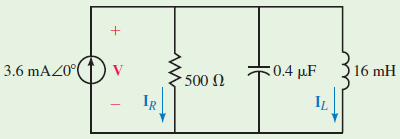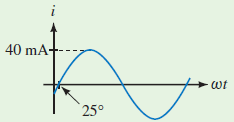# SSC JE Electrical Important MCQ Part 19

SSC JE Electrical Important MCQ PDF Part 19

1.What is the voltage across the circuit at resonance.A.1.8 V ∠0⁰

B.18 V ∠0⁰

C.0 V ∠0⁰

D.1.0 V ∠0⁰

Answer  : A (At resonance, VC=VL=VR, and so V=IR =(3.6 mA∠0⁰) (500 ∠0⁰)=1.8 V ∠0⁰)

2.Value of Irms in circuit isA.28.3 mA ∠25⁰

B.28.3 mA ∠-25⁰

C.28.3 mA ∠0⁰

D.2.83 mA ∠-25⁰

Answer  : B (i = 40 sin(ωt-25⁰) mA So Irms=0.707×Im=(0.707)×(40 mA ∠-25⁰)=28.3 mA ∠-25⁰)

3.A device that establishes an electrical connection to the earth is which of the following

A.A lightning rod

B.A bonding jumper

C.Grounded conductor

D.Grounding electrode

Answer  : D (Grounding electrode- A conducting element used to connect electrical systems or equipment to the earth)

4.Which of the following is the term for the ability of a material to allow the flow of electrons

A.Ampacity

B.Resistance

C.Current

D.Conductance

5.Which of the following symbols represents delta connectionA.a

B.b

C.c

D.d

6.A lamp that is 1000 watt, 120-volt will use the same amount of energy as a 14.4 ohm resistor at which of the following

A.1200 volts

B.240 volts

C.144 volts

D.120 volts

Answer  : D (P=VI So 1000=120*I or I=1000/120=8.33 ,Energy Same In Both So V=IR=8.33*14.4=120 V)

7.The horsepower rating of a motor is which of the following

A.The motor speed

B.The output of the motor

C.Cannot be converted to watts

D.The required input of the motor

8.The ratio of watts to volt-amperes in a DC circuit is which of the following

A.Unknown

B.Unity

C.Greater than one

D.2 :1

Answer  : B (In DC circuit P=VI,So  Watt/Volt Ampere=1)

9.The primary purpose for using thermal overload relays for polyphase induction motors is to protect against which of the following

A.Fire

B.Short circuits between phases

C.Low voltage

10.If a circuit voltage is increased and all other factors remain the same, then which of the following will change

A.Current

B.Resistance

C.Ampacity

D.All of these

11.Conductor sizes are listed as which of the following

A.Diameter

B.Area

C.AWG or circular mils

D.AWG or millimeters

Answer  : C (American Wire Gauge (AWG) is a U.S. standard for wire conductor size, The Circular Mil (CM) is a unit of area used especially to denote cross-sectional size of a wire or cable)

12.Rod and pipe grounding electrodes must not be less than which of the following lengths

A.5 feet

B.6 feet

C.8 feet

D.10 feet

Answer  : C (Rod and pipe electrodes shall not be less than 2.44 m (8 ft.) in length)

13.A protective device used for limiting surge voltages by discharging surge current and that prevents continued flow of follow current while still remaining capable of repeating these functions is considered which of the following

A.Circuit breaker

B.Fuse

B.Disconnect switch

D.Surge arrestor

14.A factor that would affect conductor ampacity is which of the following

A.The conductor length

B.Voltage

C.Temperature

D.Motor size

Answer  : C (Ampacity is defined as the maximum current, in amperes, that a conductor can carry continuously under the conditions of use without exceeding its temperature rating)

15.Which type of damping is used in MC voltmeter

A.Fluid friction damping

B.Eddy current damping

C.Air friction damping

D.Hysteresis damping

Answer  : B (Moving coil instrument uses the eddy current damping system)

16.The 2 point starter is used for starting

A.the DC Series motor

B.the DC Shunt motor

C.the DC long shunt compound motor

D.the DC short shunt compound motor

Answer  : A (The Basic function of 2 point starter is to protect DC Series motor from high starting current)

17.Ohm’s law is not applicable to

A.DC Circuits

B.Semi – Conductors

C.Resistors

D.AC Circuits

18.Which type of rotor is best suitable for high speed operation in synchronous motor

A.Salient pole rotor

B.Slip-ring rotor

C.Split-ring rotor

D.Smooth cylindrical type rotor

Answer  : D (Salient pole type rotor-Preferred only for low speed alternators of ranging from 125 r.p.m to 500 r.p.m. Cylindrical type rotor-Preferred only for high speed alternators of ranging from 1500 r.p.m to 3000 r.p.m.)

19.How many number of parallel paths will be in duplex 6 pole wave winding

A.4

B.12

C.6

D.2

Answer  : A (For wave winding parallel paths A = 2 × m, m= multiplex,So for duplex wave winding=2*2=4)

20.The value of the inductive reactance of an inductive coil when it is connected with AC supply will be

A.directly proportional to the supply frequency

B.inversely proportional to the supply frequency

C.inversely proportional to the supply voltage

D.directly proportional to the supply voltage